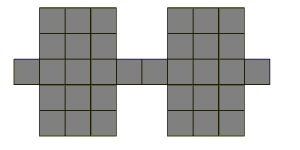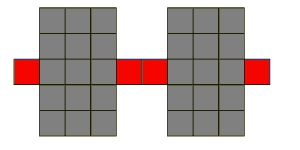﻿ MaximumWallPercent Property (LineRemoveCommand) | Leadtools.ImageProcessing.Core | Raster, Medical, Document Help
←Select platform
In This Topic ▼

# MaximumWallPercent Property

Summary
Gets or sets the maximum number of wall slices (expressed as a percent of the total length of the line) that are allowed.
Syntax
C#
Objective-C
C++/CLI
Java
Python
``public int MaximumWallPercent { get; set; } ``
``@property (nonatomic, assign) NSInteger maximumWallPercent; ``
````public int getMaximumWallPercent(); `
`public void setMaximumWallPercent( `
`   int intValue `
`); ````
````public: `
`property int MaximumWallPercent { `
`   int get(); `
`   void set (    int ); `
`} ````
``MaximumWallPercent # get and set (LineRemoveCommand) ``

#### Property Value

The maximum number of wall slices (expressed as a percent of the total length of the line) that are allowed. A line consists of wall slices and non-wall slices (see description of Wall). This property specifies the maximum number of wall slices (expressed as a percent of the total length of the line) that are allowed. Lines that have a wall percent that is larger than maximumWallPercent will not be removed. For example, consider the line below that is 10 pixels in length, and 1 pixel in height in some places, and five pixels in height in other places.Setting MinimumLineLength to 10 pixels and Wall to four pixels will identify the line below as a candidate for removal. Note that the wall percent for this line is 60 percent (because six of ten line slices are walls). If MaximumWallPercent is 80 then the line will be removed (actually, the pixels marked as red will be removed). If MaximumWallPercent is 30, then the line will not be removed.Example
C#
````using Leadtools; `
`using Leadtools.Codecs; `
`using Leadtools.ImageProcessing.Core; `
` `
`public void LineRemoveCommandExample() `
`{ `
`   // Load an image `
`   RasterCodecs codecs = new RasterCodecs(); `
`   codecs.ThrowExceptionsOnInvalidImages = true; `
` `
`   RasterImage image = codecs.Load(Path.Combine(LEAD_VARS.ImagesDir, "Clean.tif")); `
` `
`   // Prepare the command `
`   LineRemoveCommand command = new LineRemoveCommand(); `
`   command.LineRemove += new EventHandler<LineRemoveCommandEventArgs>(LineRemoveEvent_S1); `
`   command.Type = LineRemoveCommandType.Horizontal; `
`   command.Flags = LineRemoveCommandFlags.UseGap; `
`   command.GapLength = 2; `
`   command.MaximumLineWidth = 5; `
`   command.MinimumLineLength = 200; `
`   command.MaximumWallPercent = 10; `
`   command.Wall = 7; `
`   command.Run(image); `
` `
`} `
` `
`private void LineRemoveEvent_S1(object sender, LineRemoveCommandEventArgs e) `
`{ `
`   MessageBox.Show("Row Col " + "( " + e.StartRow.ToString() + ", " + e.StartColumn + " )" + `
`      "\n Length " + e.Length.ToString()); `
`   e.Status = RemoveStatus.Remove; `
`} `
` `
`static class LEAD_VARS `
`{ `
`   public const string ImagesDir = @"C:\LEADTOOLS22\Resources\Images"; `
`} ````
Requirements